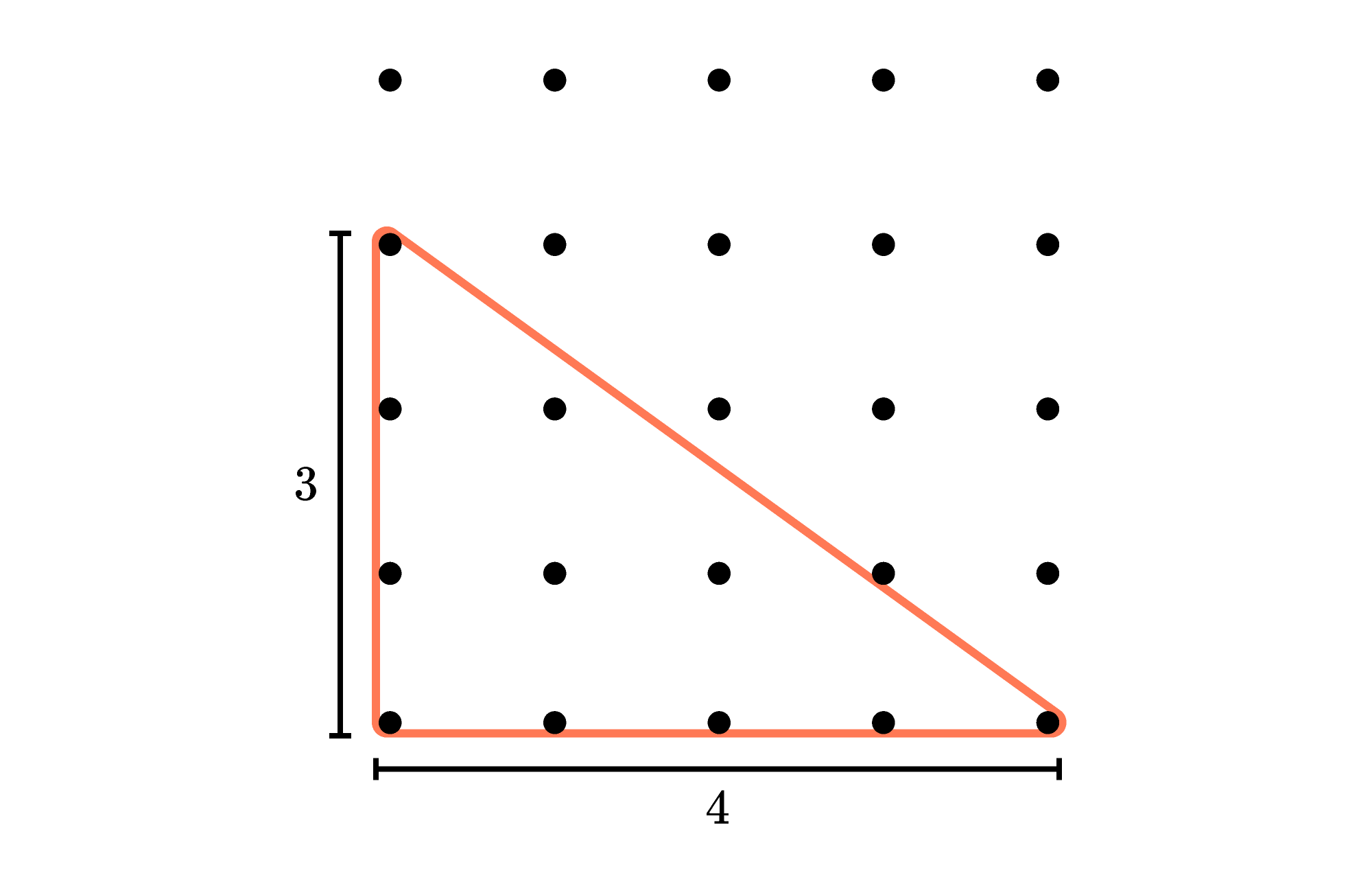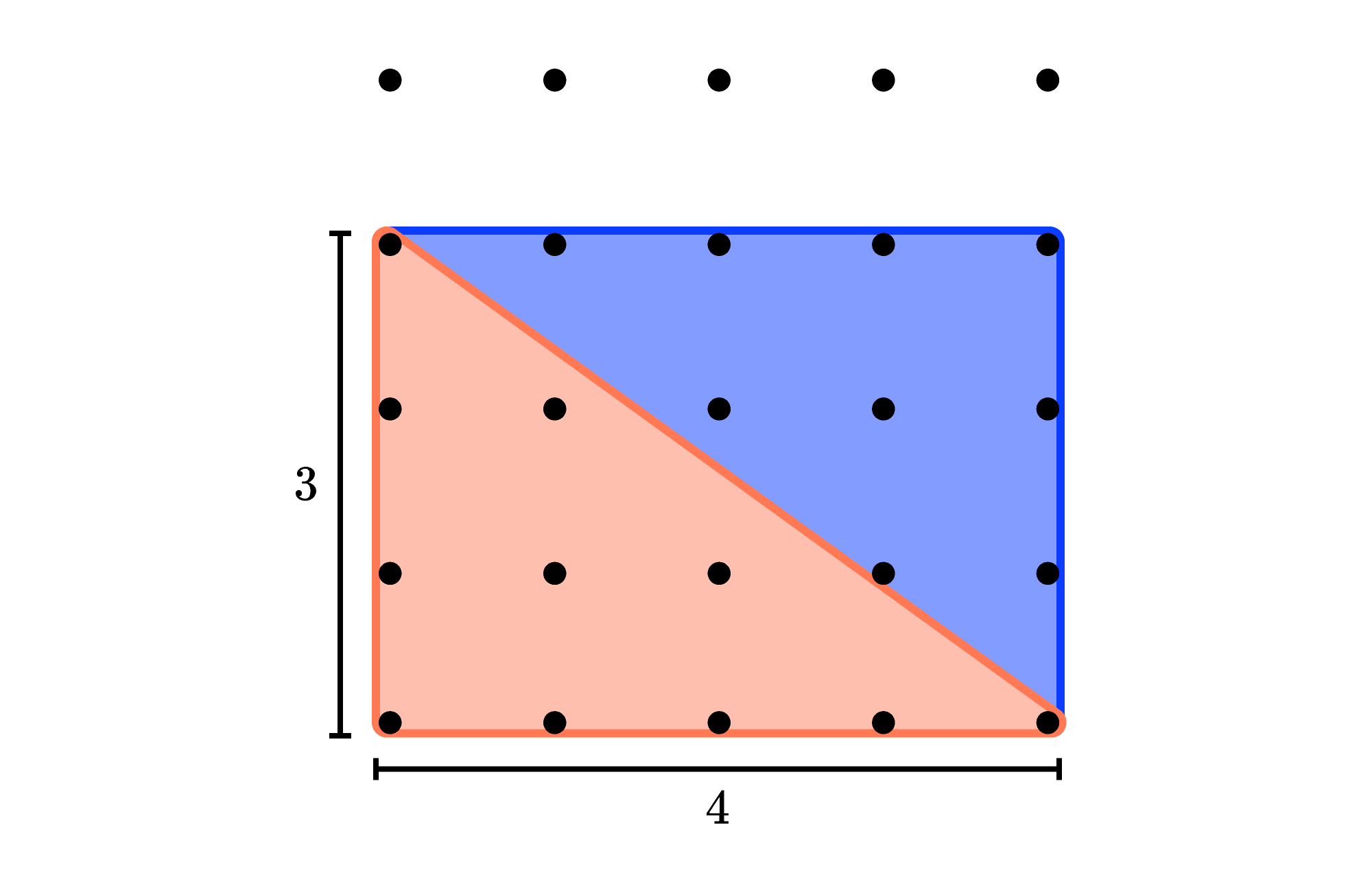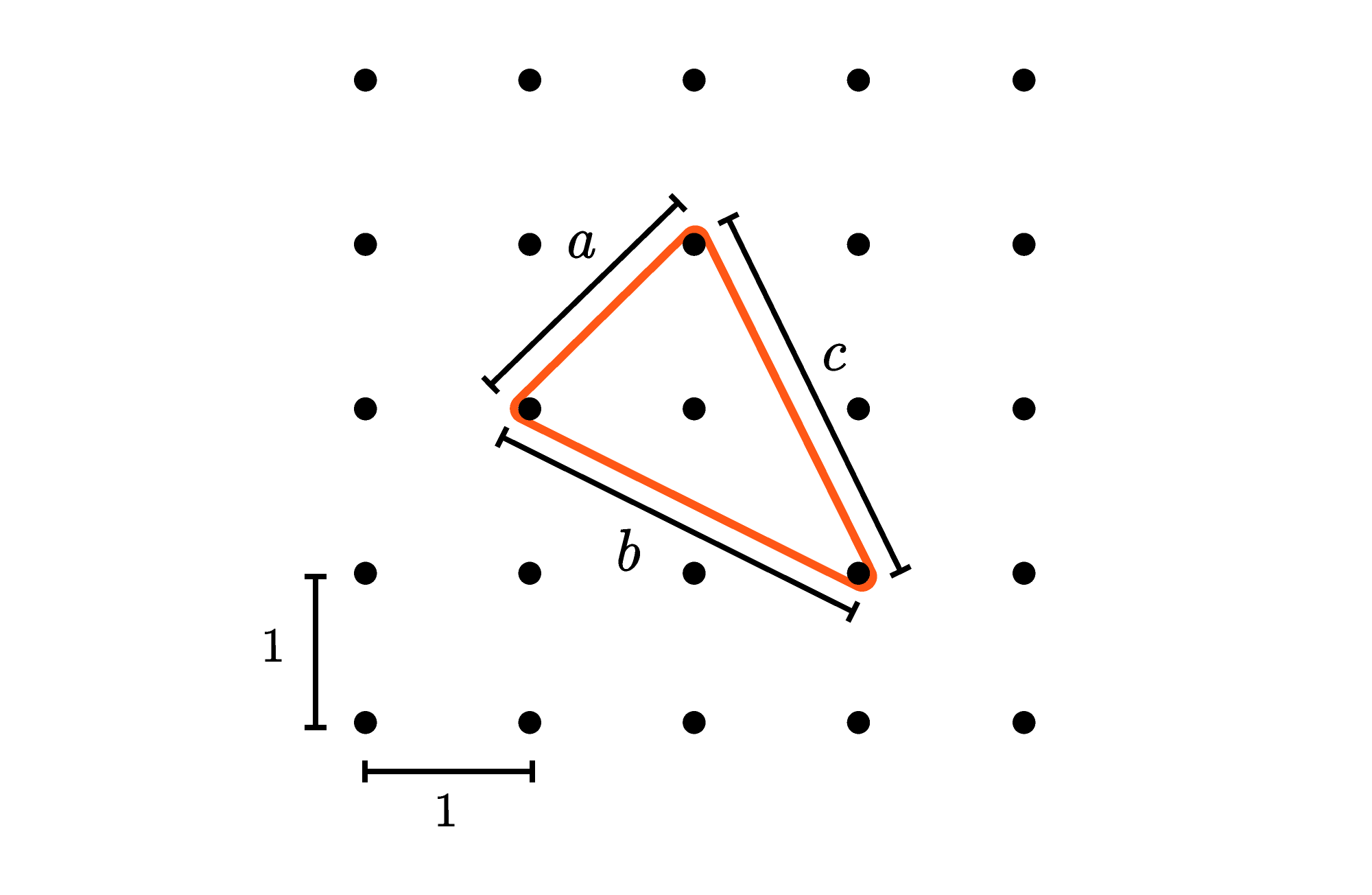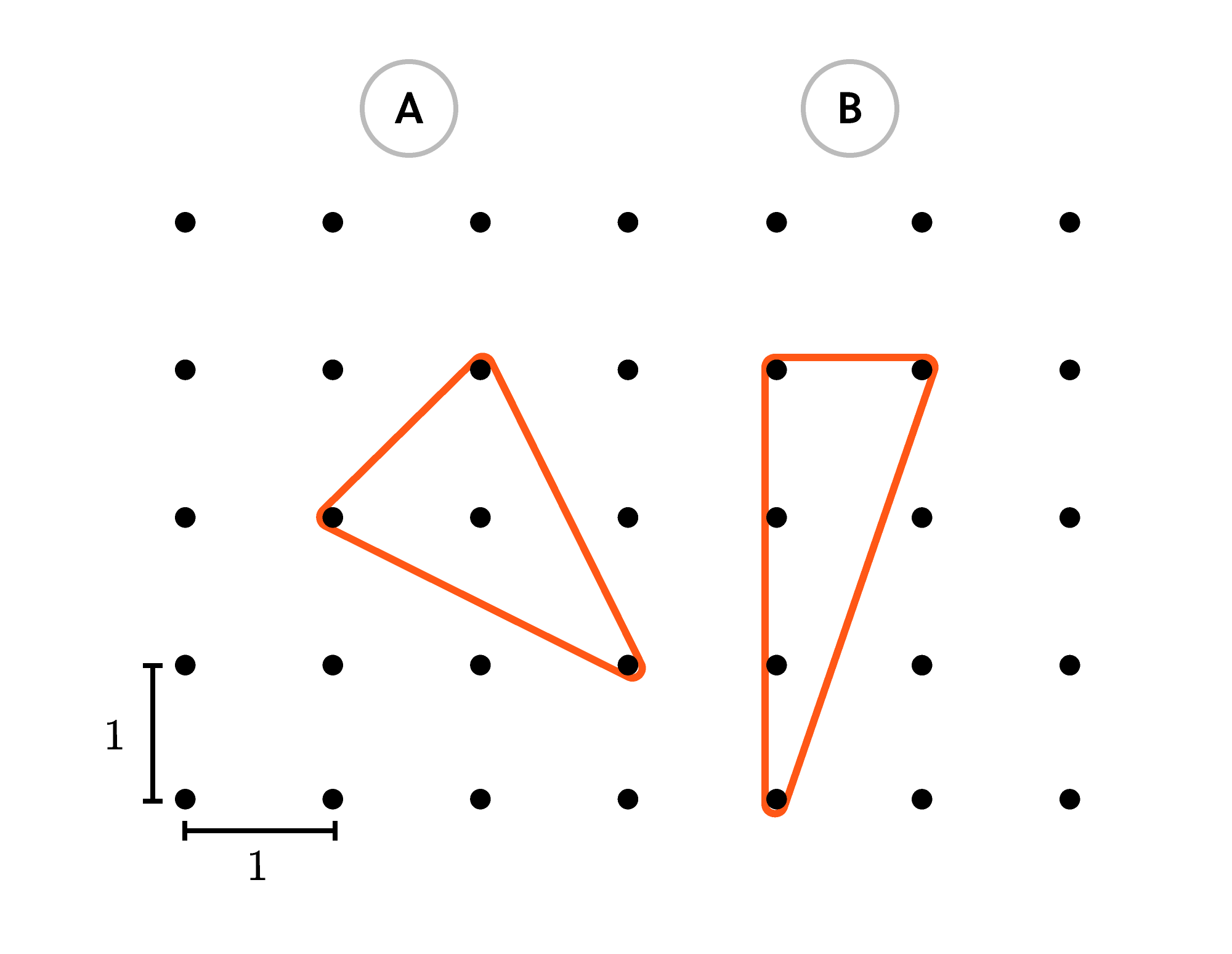Back

## Not a Pythagorean Triple

A square lattice is a regular arrangement of points in a grid pattern such as the one below.Each of the points in this lattice is $1$ unit away from the points that are directly above, below, and to the sides of it, so this triangle has two legs that measure $3$ and $4,$ respectively.

Additionally, since this is a right triangle, we can find the length of the third side using the Pythagorean theorem:

$\sqrt{3^2 + 4^2} = \ ?$

The third side length of this particular triangle is $5,$ meaning that this triangle's three sides form a Pythagorean triple: a set of three integers $(3, 4,$ and $5)$ that fit into the Pythagorean theorem: $3^2 + 4^2 = 5^2.$

The triangle's area is also an integer. Any triangle's area is equal to one half of the length of its base times its height. In this case, the area is $\frac{3 \times 4}{2} = 6,$ and the application of the area equation can be visualized by inscribing the triangle inside of a $3\times 4$ rectangle that it fills exactly half of.But what if a triangle's edges aren't all integer lengths? Can such a triangle still have an integer area? Also, is it possible for a triangle with no integer side lengths to still have three vertices that all coincide with points in a square lattice?

With respect to that second question, let's think about how we might try to make such a triangle. Note that any sides of the triangle that are purely horizontal or vertical will definitely have integer lengths, so we'll need to pick points that aren't in the same rows or columns. Here's one possibility.To be certain all of these diagonal lines have non-integer lengths, we can calculate all three of them using the Pythagorean theorem:

• $a: 1^2 + 1^2 = a^2\implies$ length: $\sqrt{2}$
• $b: 1^2 + 2^2 = b^2\implies$ length: $\sqrt{5}$
• $c: 1^2 + 2^2 = c^2\implies$ length: $\sqrt{5}.$

So we've successfully created a triangle with three non-integer sides, and it turns out that our triangle is also isosceles. In today's challenge, the goal is to find its area. This can be accomplished by using the Pythagorean theorem to find base and height measures for the triangle. However, there's also an elegant way to use "negative space" to find the triangle's area. Or maybe you'll come up with another method for figuring out the area — if you do, please share it in the problem's comment thread.

# Today's Challenge

Which of these two lattice triangles has the larger area?×